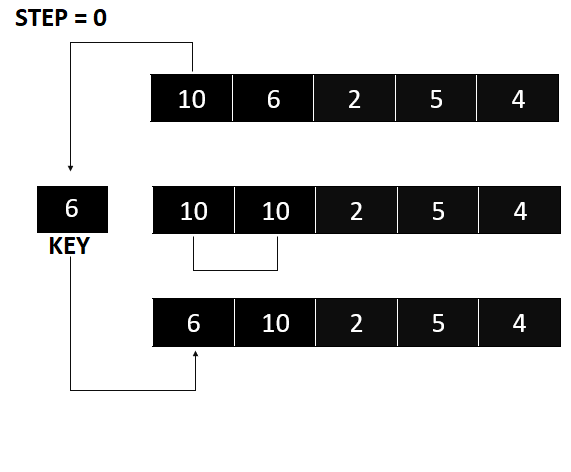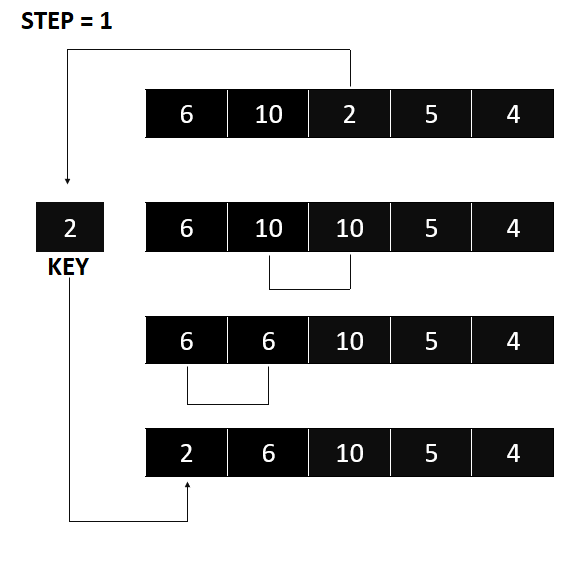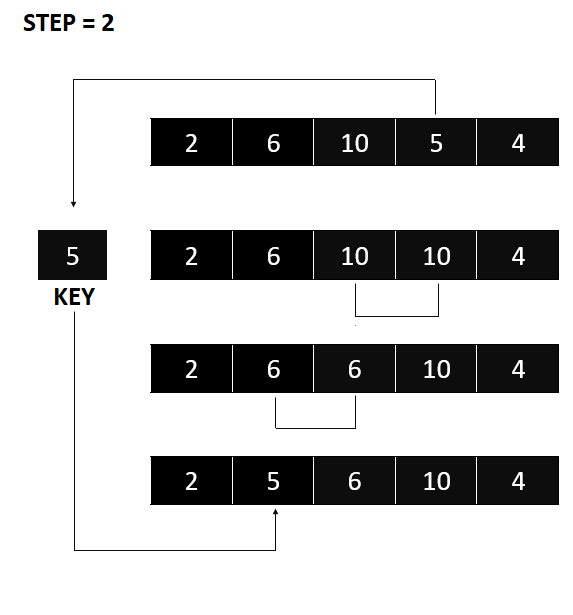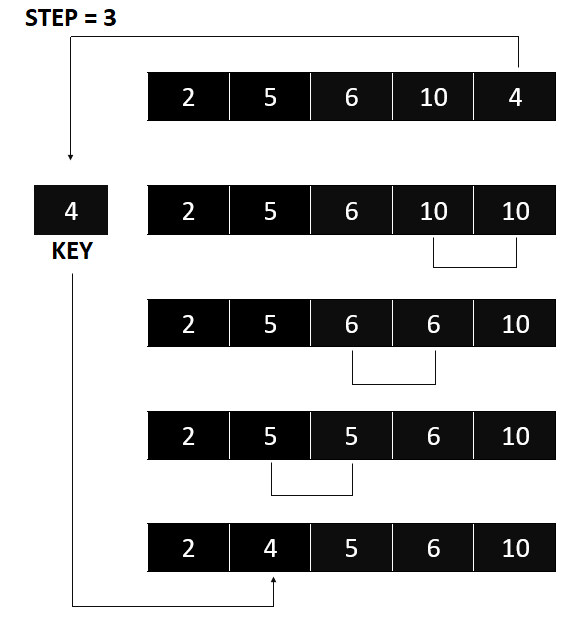# Insertion Sort In Java With Example Code

In this Insertion Sort In Java tutorial, we will discuss all the things you need to know about this sort algorithm, as well as how it is implemented. This tutorial could be one of your fundamental learning guides on your journey as a Java developer.

## What Is Insertion Sort In Java?

The Insertion Sort In Java Program is a simple sorting method that provides for efficient, one-at-a-time sorting of an array. By in-place sorting, we imply that the original array is changed without the requirement for temporary structures.

Insertion sort operates in the same way that we sort cards in our hands when playing a card game.

We select an unsorted card after assuming the first card has already been sorted. If the unsorted card is larger than the card in hand, it goes to the right; otherwise, it goes to the left. Other unsorted cards are taken and placed in their proper locations in the same manner.

Insertion sort employs a similar strategy.

We’ll look at how to implement this sorting technique in Java.

## Visualizing the sorting procedure

Consider the following array, which we need to sort.

• The first element of the array is assumed to be sorted. Take the second element and store it separately in the key.

Compare the first element to the key. If the first element is bigger than key, the first element is placed in front of key.Insertion Sort – If the first element is greater than key, then key goes in front of the first element.
• The first two elements have now been sorted.

Look at the third element and compare it to the ones to its left. Put it right behind the thing that was smaller than it. If there’s nothing smaller than it, put it at the start of the array.Insertion Sort – Put the number 2 at the beginning
• In the same way, put every unsorted element in its right position.Insertion Sort – Put 5 behind 2Insertion Sort – Put 4 behind 2, and the array will get sorted perfectly

## Insertion Sort In Java Sample Code

You can run this code in your code editor if you already downloaded it to your laptop or PC, but if you don’t, you can download it. Here’s the link. NetBeans IDE, but if you wish to run this code online, we also have an online compiler in Java. Here’s the link. Java Online Compiler

We also put the Java online compiler below. Just scroll down so that you can easily test or run the Insertion Sort source code in order to learn more about this sort algorithm.

## Sample Java Code Using Scanner.

Scanner is a class in the Java Utilities package that is used to get the input of primitive types like int, double, etc., and strings. It is the easiest way to read input into a Java program.

```package insertionsort_in_java;

import java.util.Scanner;
public class InsertionSort_in_Java {

public static void Sort(int a[]) {
int n = a.length, i, j, p, temp;
for (i = 1; i < n; i++) {

for (j = i - 1; j >= 0 && a[j + 1] < a[j]; j--) {
temp = a[j + 1];
a[j + 1] = a[j];
a[j] = temp;

}

}
}

public static void printarray(int a[]) {
for (int i = 0; i < a.length; i++) {

System.out.print(a[i] + " ");
}

}

/**
* @param args the command line arguments
*/
public static void main(String[] args) {
int n, res, i;
Scanner s = new Scanner(System.in);
System.out.print("Enter number of elements in the array:");
n = s.nextInt();
int a[] = new int[n];
System.out.println("Enter " + n + " elements ");
for (i = 0; i < n; i++) {
a[i] = s.nextInt();
}

System.out.println("elements in array ");
printarray(a);
Sort(a);
System.out.println("\nelements after sorting");
printarray(a);

}
}
```

If you wish to use our online compiler to run this Insertion Sort source code, simply copy all of the code and paste it there. Here’s the URL to the page. The Java Online Compiler is a tool that allows you to compile Java code online

## Insertion Sort Example Output Using Scanner

```Enter number of elements in the array:Enter 5 elements
elements in array
10 6 2 5 4
elements after sorting
2 4 5 6 10 ```

## Test Your Insertion Sort Code

Anyway, if you want to level up your programming knowledge, especially Java, try this new article I’ve made for you Best Java Projects With Source Code For Beginners Free Download 2021.

## Conclusion

So far, we’ve already discussed the Insertion Sort algorithm for sorting in Java. We also looked at the algorithm and a detailed example of how to use the Selection Sort technique to sort an array and using scanner. Then we put the Java program to work on the Selection Sort. I hope this Selection Sort tutorial helps you a lot in learning Java development.

Bubble Sort

Selection Sort

## Inquiries

If you have any questions or suggestions about the Insertion Sort, please feel free to leave a comment below.